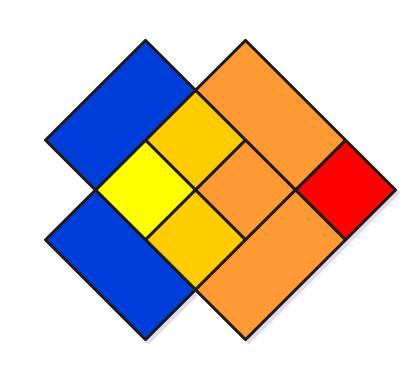CSCI 480: Intro' to Computer Graphics

Fall 2011 - Final Exam

12/7/11, 6:30-8:00 PM

``

Name: ____________________________________

Student ID: ______________________________

1   10
2   10
3   10
4   10
5   10
6   10
7   10
8   10
9   10
10   10
Bonus   5
Total   105

Have fun, good luck!

Question 1 [5+5 = 10 points]

Why does 3D (ie XYZ) computer graphics need 4x4 matrices (and not 3x3) for rotation, translation and scale?

A. Because the translation matrix cannot be expressed as a 3x3, it can only be 4x4. Because of the need to multiply (compose) matrices, we promote the others (R, S) to 4x4 as well.

Given a rotation matrix `R(theta)` (about X or Y or Z) that rotates a point `p` clockwise, what are two ways, other than inverting `R`, to rotate `p` the other way (ie. counter-clockwise)?

A.
a. do `R(-theta)`
b. use `R^T` (transpose).

Question 2 [5+5= 10 points]

What would happen if, before using it to calculate shadows, all the pixel values in a depth map were to be brightened by a fixed amount (ie. same brightening is applied throughout the map)?

A. Shadow placement would be affected, ie incorrect-looking shadows would result (eg. a shadow might creep up over a surface that is casting the shadow; conversely, the shadow might become detached from the shadow casting surface, in a contact situation where the shadow needs to be adjacent to the shadow caster.

Filtering is a way to create soft-edged shadows. In what should the following be done - depth comparison, filtering, and why?

A. Depth-compare, then filter. See class notes for a diagram that illustrates it both ways (one good, other, bad).

Question 3 [2*5= 10 points]

Name one OpenGL call that achieves the following (ie name 5 calls total):

• sets vertex color:
• sets up a camera:
• draws a line:
• turns a light on:
• switches between camera and model transformation stacks:

A. glVertex{2f,2i,2d,3f,3i.3d}, gluPerspective, glOrtho, etc., glBegin(GL_LINES), glEnable(GL_LIGHT0 etc., glMatrixMode()

Question 4 [2*5= 10 points]

What is the advantage of using splines to create curves, as opposed to using explicit curve equations?

A. Precise control over curve shape.

Particle-systems employ a huge number of particles to create effects such as sparks, spray, etc. But particles are zero-dimensional entities, and hence can't be textured. How do we usually overcome this in CG?

A. Use sprites instead, which are camera-facing rectangles that are instanced at each particle position.

Question 5 [10 points]

Shown below is a character, with relatively few 'hero hairs' placed on her head. From these, how is head full of hair (millions of strands) generated during rendering? Be specific, ie. discuss the technique using appropriate equations, and an illustration.

A. Barycentric (triangle coord system) interpolation is used, where for each hair/fur attribute (eg. color), the hero values (which always lie on triangle vertices, ie. the hero hair locations are triangulated first) are interpolated according to barycentric weights (which sum to 1.0). In other words, it is all about weighted interpolation, using triplets of local weights.

Question 6 [10 points]

Scan-converting polygons is a classic way to render them. A single polygon produces an unambiguous, straightforward render. But in a scene with dozens of objects that contain thousands/millions of polygons at varying depths from the camera, what keeps these polygon scans from interfering with each other to create a 'jumbled' image? Discuss the most common solution for this, in detail.

A. Use z-buffering. See notes for more.

Question 7 [2*5= 10 points]

In CG, what is 'noise()', and why is it useful?

A. noise() is a spatially continuous and varying function, that outputs a single float/double value at each 1D, 2D, 2D, 4D or for that matter, nD point.

From noise(), how is turbulence() derived?

A. See notes - we scale and add noise() over a range of frequencies and amplitudes.

Question 8 [2.5 + 3*2.5= 10 points]

What is the origin of GPUs?

A. GPUs evolved from OpenGL accelerator cards, which were based on chipsets that implemented the OpenGL pipeline in hardware.

Current GPUs are supposed to be 'third generation'. What characterizes the first, second and third generations of GPUs?

A.
Gen1: programmable vertex processors
Gen2: above, plus programmable pixel processors
Gen1: general-purpose, programmable shader processors (no specific allocations for vertex vs pixel processing)

Question 9 [4*2.5 = 10 points]

"Computer animation" (in 3D CG) can be achieved in a number of ways. Name four different animation techniques, and describe each briefly (in a sentence or two).

A. See notes: FK, IK, mocap, procedural (script-based), behavioral (higher level, 'AI' based)..

Question 10 [2.5 + 3*2.5= 10 points]

'Radiosity rendering' is one form of GI (global illumination). How does it work? Discuss using an illustration or two.

A. See notes - briefly, radiosity involves calculation of 'form factors' (extent to which emissive surfaces are oriented towards nearby receivers), and using these in conjunction with emissivity values in order to 'bleed' color/shading on to adjacent receiver surfaces.

In addition to radiosity, there are other techniques that make up the umbrella of GI techniques. Name three of them.

A. Ambient occlusion, caustics, subsurface scattering.

Bonus question [5 points]

How many squares are visible in the following diagram?A. 11